#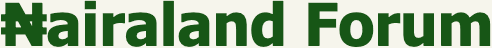Welcome, Guest: Join Nairaland / LOGIN! / Trending / Recent / New
Stats: 2,738,517 members, 6,493,203 topics. Date: Friday, 17 September 2021 at 05:38 AM

## Plz Help!!urgent Need Of Solutions To Some Maths Problems,thanks As U Help - Education - Nairaland

 Plz Help!!urgent Need Of Solutions To Some Maths Problems,thanks As U Help by Nobody: 3:12pm On Jun 12, 2019 Good afternoon people, am really in need of solutions to some questions and I'll be glad if my thread is being moved to FPLalasticlala, Mynd44, thanks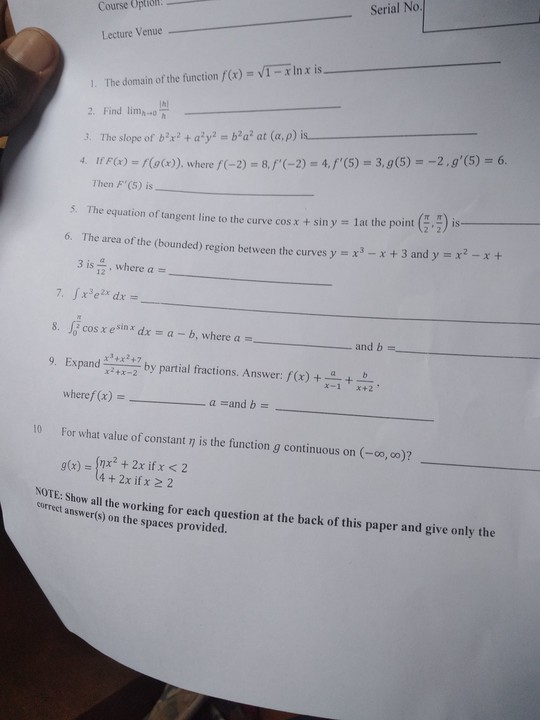Re: Plz Help!!urgent Need Of Solutions To Some Maths Problems,thanks As U Help by Regiomontanus(m): 5:36pm On Jun 12, 2019 @cole01 This exercise requires a good knowledge of calculus. Here are the answers. 1) The domain is the interval (0, 1].2) The limit does not exist. 3) The gradient at (a, ρ) is -b²/aρ4) F'(x) = 245) The slope is undefined. Either it has a vertical tangent line of none at all. I will investigate the behaviour of the function later to conclude which is it.Edit: the tangent line doesn't exist. 6) a = 17) ½x³e2x - ¾x²e2x + ¾xe2x - ⅜e2x + C8} a = e and b = 1, e is the Euler's constant ie. the base of the natural logarithmic function.9) f(x) = x, a = 3 & b = -110) n = 1 Re: Plz Help!!urgent Need Of Solutions To Some Maths Problems,thanks As U Help by Nobody: 6:00am On Jun 13, 2019 Regiomontanus:@cole01 This exercise requires a good knowledge of calculus. Here are the answers. 1) The domain is the interval (0, 1].2) The limit does not exist. 3) The gradient at (a, ρ) is -b²/aρ4) F'(x) = 245) The slope is undefined. Either it has a vertical tangent line of none at all. I will investigate the behaviour of the function later to conclude which is it.Edit: the tangent line doesn't exist. 6) a = 17) ½x³e2x - ¾x²e2x + ¾xe2x - ⅜e2x + C8} a = e and b = 1, e is the Euler's constant ie. the base of the natural logarithmic function.9) f(x) = x, a = 3 & b = -110) n = 1 RegiomontanusThank you very much sir, plz can I just get the picture of the solvings, I remain grateful Re: Plz Help!!urgent Need Of Solutions To Some Maths Problems,thanks As U Help by Nobody: 9:29pm On Jun 13, 2019 [quote author=Regiomontanus post=79267919]@cole01 This exercise requires a good knowledge of calculus. Here are the answer please I've been waiting Sir, really need it urgently Re: Plz Help!!urgent Need Of Solutions To Some Maths Problems,thanks As U Help by Regiomontanus(m): 9:45pm On Jun 13, 2019 cole01: please I've been waiting Sir, really need it urgently I have been busy. I will send them very soon. I need to eat first. Re: Plz Help!!urgent Need Of Solutions To Some Maths Problems,thanks As U Help by Nobody: 10:10pm On Jun 13, 2019 Regiomontanus: I have been busy. I will send them very soon. I need to eat first. ok sir, thanks for ur reply Re: Plz Help!!urgent Need Of Solutions To Some Maths Problems,thanks As U Help by Nobody: 8:23am On Jun 14, 2019 Regiomontanus: I have been busy. I will send them very soon. I need to eat first. Sir I've been waiting, submitting by 10am Re: Plz Help!!urgent Need Of Solutions To Some Maths Problems,thanks As U Help by Regiomontanus(m): 9:12am On Jun 14, 2019 cole01:Sir I've been waiting, submitting by 10am Sorry, my day has been tight.1) if f(x) = g(x)h(x), then the domain of f(x) is the intersection of the domains of g(x) and h(x). The domain of g(x) = (1 - x)½ is (-∞, 1] and the domain of h(x) = ln x is the set of all positive numbers ie. the interval (0, ∞). Therefore the domain of f(x) = g(x)h(x) = (1 - x)½ ln x is (0, 1].Typing... cole01 Re: Plz Help!!urgent Need Of Solutions To Some Maths Problems,thanks As U Help by Regiomontanus(m): 9:20am On Jun 14, 2019 2) the limit doesn't exist because on both sides of 0, the function approaches two different numbers (-1 from the left and 1 from the right).3) Find dy/dx for b²x² + a²y² = b²a² using implicit differentiation. We have 2b²x + 2a²y(dy/dx) = 0. Hence, dy/dx = -b²x/a²y. @ (a, p), dy/dx = -b²/apTyping... cole01 Re: Plz Help!!urgent Need Of Solutions To Some Maths Problems,thanks As U Help by Regiomontanus(m): 9:30am On Jun 14, 2019 4) if F(x) = f(g(x)) then by the chain rule of differentiation, F'(x) = f'(g(x))g'(x). So F'(5) = f'(g(5))g'(5) if g(5) = -2 then f'(g(5)) = f'(-2) = 4 and g'(5) = 6. Therefore, F'(5) = (4)(6) = 24typing ...cole01 Re: Plz Help!!urgent Need Of Solutions To Some Maths Problems,thanks As U Help by Regiomontanus(m): 9:44am On Jun 14, 2019 5) Using implicit differentiation to find dy/dx for cos x + sin y = 1 leads to -sin x + cos y (dy/dx) = 0 Therefore dy/dx = sin x / cos y , at (π/2, π/2) dy/dx = 1/0 = udf The tangent line is nonexistent.6) The points of intersection of both graphs are x = 0 and x = 1 and on the interval [0, 1], y1 = x2 - x + 3 is greater than or equal to y2 = x3 - x + 3. So integrate y1 - y2 = x2 - x3 from 0 to 1 and you will get 1/12. therefore a = 1typing...cole01 Re: Plz Help!!urgent Need Of Solutions To Some Maths Problems,thanks As U Help by Brunicekid(m): 9:47am On Jun 14, 2019 Mathematics, the father of all subjects... Argue with your keypads.Meanwhile, i will try and solve the questions. 1 Like 1 Share Re: Plz Help!!urgent Need Of Solutions To Some Maths Problems,thanks As U Help by Regiomontanus(m): 9:56am On Jun 14, 2019 7) You will have to use integration by parts repeatedly to get the answer. I can,t write them as I am on a bus. 8} An antiderivative of the integrand f(x) = (cos x)esin x is F(x) = esin x because F'(x) = f(x). Using the first fundamental theorem of calculus, the definite integral is esin π/2 - esin 0 = e1 - e0 = e - 1.Therefore a = e and b = 1 typing...cole01 Re: Plz Help!!urgent Need Of Solutions To Some Maths Problems,thanks As U Help by Regiomontanus(m): 10:11am On Jun 14, 2019 sorry that this is coming too late,9) using long division (x3[sup]+x[sup]2+7)/(x2+x-2) can be written as x + [(2x+7)/(x2+x-2)] Apply the method of partial fraction to the proper rational expression ,(2x+7)/(x2+x-2) = (2x+7)/[(x-1)(x+2)] = A/(x-1) + B/(x+2) Multiply both sides with (x-1)(x+2), 2x+7 = A(x+2) + B(x-1). If x = 1 , A = 3 and if x = -2, B = -1. So (x3+x2+7)/(x2+x-2) = x + 3/(x-1) + -1/(x+2).therefore f(x) = x , A = 3 and B = -1typing... Re: Plz Help!!urgent Need Of Solutions To Some Maths Problems,thanks As U Help by Regiomontanus(m): 10:13am On Jun 14, 2019 cole01, I am sorry for not sending the solutions on time. I was busy and exhausted.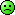Re: Plz Help!!urgent Need Of Solutions To Some Maths Problems,thanks As U Help by Regiomontanus(m): 10:18am On Jun 14, 2019 10) nx2 + 2x = 4 + 2x at x = 2 to ensure continuity. 4n + 4 = 8. therefore n = 1 the end. cole01 1 Like Re: Plz Help!!urgent Need Of Solutions To Some Maths Problems,thanks As U Help by Nobody: 9:53pm On Jun 14, 2019 Regiomontanus:10) nx2 + 2x = 4 + 2x at x = 2 to ensure continuity. 4n + 4 = 8. therefore n = 1 the end. cole01Thanks Sir, am so so grateful for every thing,thanks Re: Plz Help!!urgent Need Of Solutions To Some Maths Problems,thanks As U Help by Regiomontanus(m): 7:09am On Jun 15, 2019 cole01:Thanks Sir, am so so grateful for every thing,thanks Were you able to copy them and support? In case you have any maths questions, mention me. If I can solve them, I will and I will send the workings and answers as fast as possible.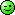1 Like Re: Plz Help!!urgent Need Of Solutions To Some Maths Problems,thanks As U Help by Nobody: 12:29pm On Jun 29, 2019 Regiomontanus: Were you able to copy them and support? In case you have any maths questions, mention me. If I can solve them, I will and I will send the workings and answers as fast as possible.1.The total area under the normal distribution curve equals?? Regiomontanus Thanks once again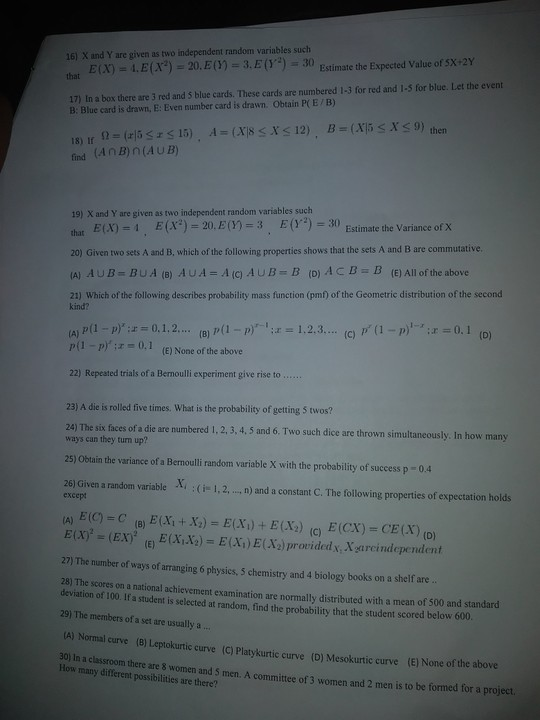Re: Plz Help!!urgent Need Of Solutions To Some Maths Problems,thanks As U Help by Regiomontanus(m): 9:15pm On Jun 29, 2019 cole01:1.The total area under the normal distribution curve equals?? Regiomontanus Thanks once again These questions are beyond my limit. I am not informed in probability and statistics. Re: Plz Help!!urgent Need Of Solutions To Some Maths Problems,thanks As U Help by Nobody: 8:29pm On Jul 01, 2019 Regiomontanus: These questions are beyond my limit. I am not informed in probability and statistics. Ok brother,Thank you sir for helping, I appreciate Re: Plz Help!!urgent Need Of Solutions To Some Maths Problems,thanks As U Help by Cmanforall: 10:38pm On Jul 01, 2019 Regiomontanus: Were you able to copy them and support? In case you have any maths questions, mention me. If I can solve them, I will and I will send the workings and answers as fast as possible.This guy go dey exam hall, snap picture send you. You solve within a particular time frame.My advice: He should attend classes. Practice exercises. When faced with challenging questions, he can then bring them here. You can solve questions for him, don't get me wrong. But that time limit for showing your calculations should not be encouraged.#IStandAgainstExamMalpractice Re: Plz Help!!urgent Need Of Solutions To Some Maths Problems,thanks As U Help by NPFofficer: 11:32pm On Sep 19, 2020 cole01:Good afternoon people, am really in need of solutions to some questions and I'll be glad if my thread is being moved to FPLalhanksYoung man, I see your hand for that thread okay. You no know say people wey get mouth dey read am abi? I am from division E, akpehe. See I'm not begging you to cooperate okay? But if you love your life and your future just do the needful. Send small cut out of that money or from your personal money so we can close the case because I don check your data base, I've gotten your address and profile already. Let me know your decision before 8Am tomorrow. If you fail to cooperate, I will send my boys to carry you and your mama. They go kill you throway inside bush and nobody will know . Or better still we will carry you to station and frame you up for armed robbery and murder. So just coporate okay? It's for your own good. Before 8AM tomorrow give me feedback. Re: Plz Help!!urgent Need Of Solutions To Some Maths Problems,thanks As U Help by NPFofficer: 7:02am On Sep 20, 2020 cole01:Good afternoon people, am really in need of solutions to some questions and I'll be glad if my thread is being moved to FPiclala, M44, thanksSo despite my advice you refused to coporate abi? Martin's, you're a dead man walking. We will get you arrested and frame you up for murder and armed robbery. So those boys wey die for Ebete last week na you kill them abi?. Your life don finish. I still give you grace o, till 12PM else , your doom is assured. Me and my team will frustrate your life and that of your family.